# Height of a Binary Tree

## Introduction

A binary tree is an important data structure with applications in computer science, from operating systems to building servers. Using binary trees can reduce the complexity of various problems to a large extent.

The depth of a tree node is equal to the number of edges from the node to the tree's root node. A root node has a depth of 0.

The height of a tree node is equal to the number of edges on the longest path from the node to a leaf. A leaf node has a height of 0.

In this blog, we will be covering a very common question on Binary trees: How can we calculate the height of a binary tree?

Let’s understand it using an example.

Consider the binary tree given below: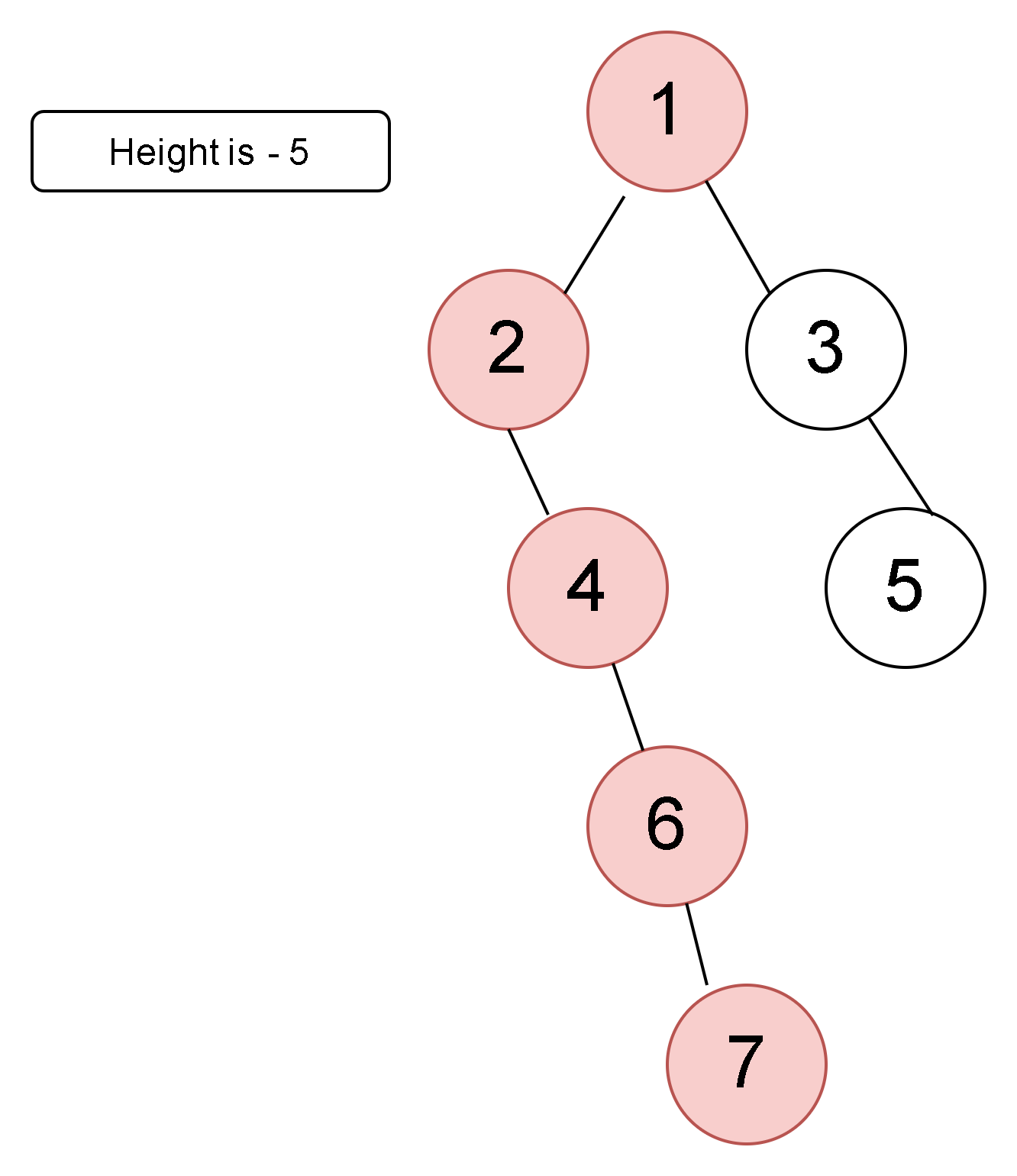In the tree given above,

Height - the height of a root node is 5 since the longest path from the root node to any of the leaf nodes is 5.

Depth - the depth of the root node will be 0 since we are at the root node.

The longest path is coloured, i.e. 1->2->4->6->7.

The path for getting the height of the binary tree will be traversed as shown below :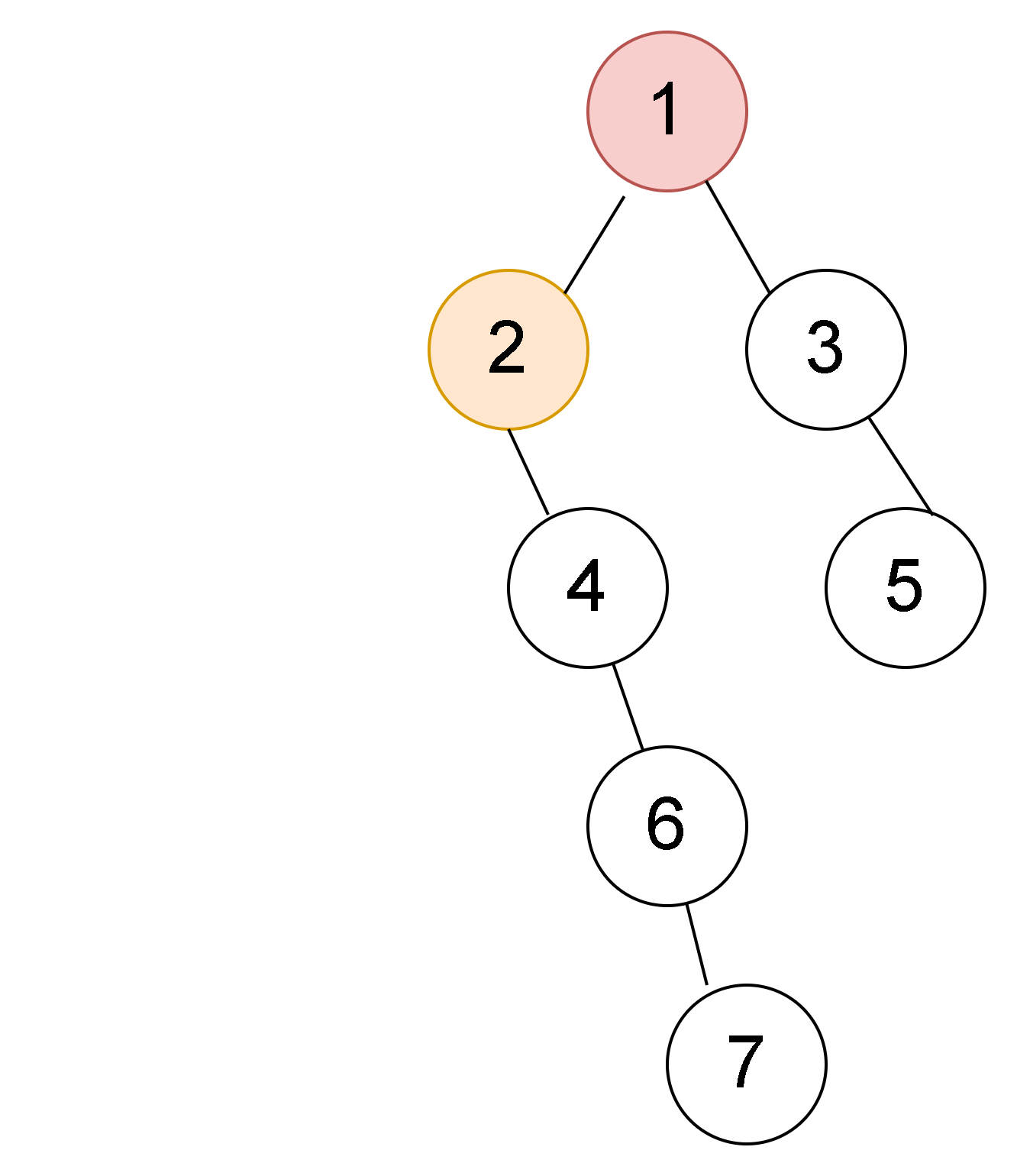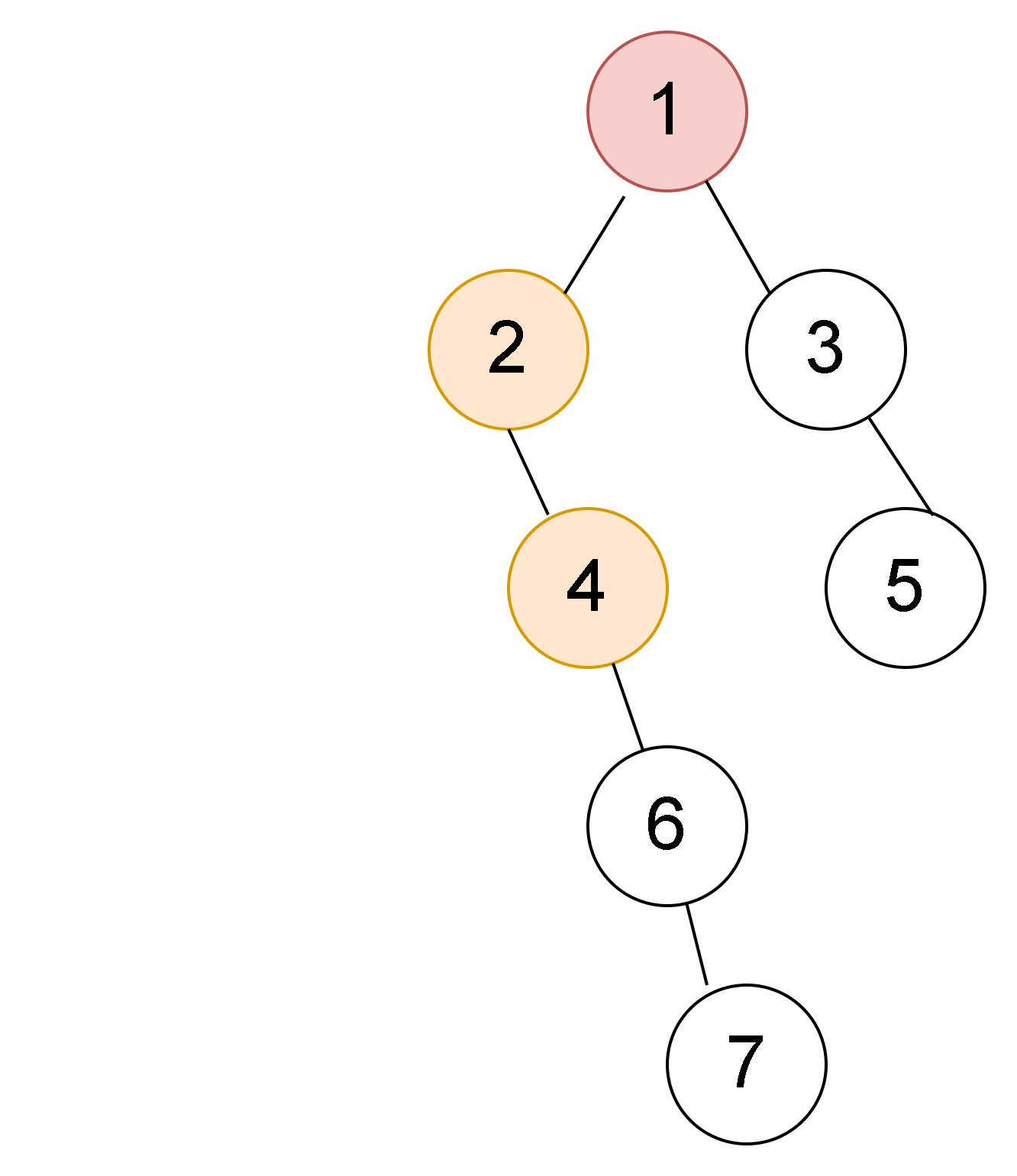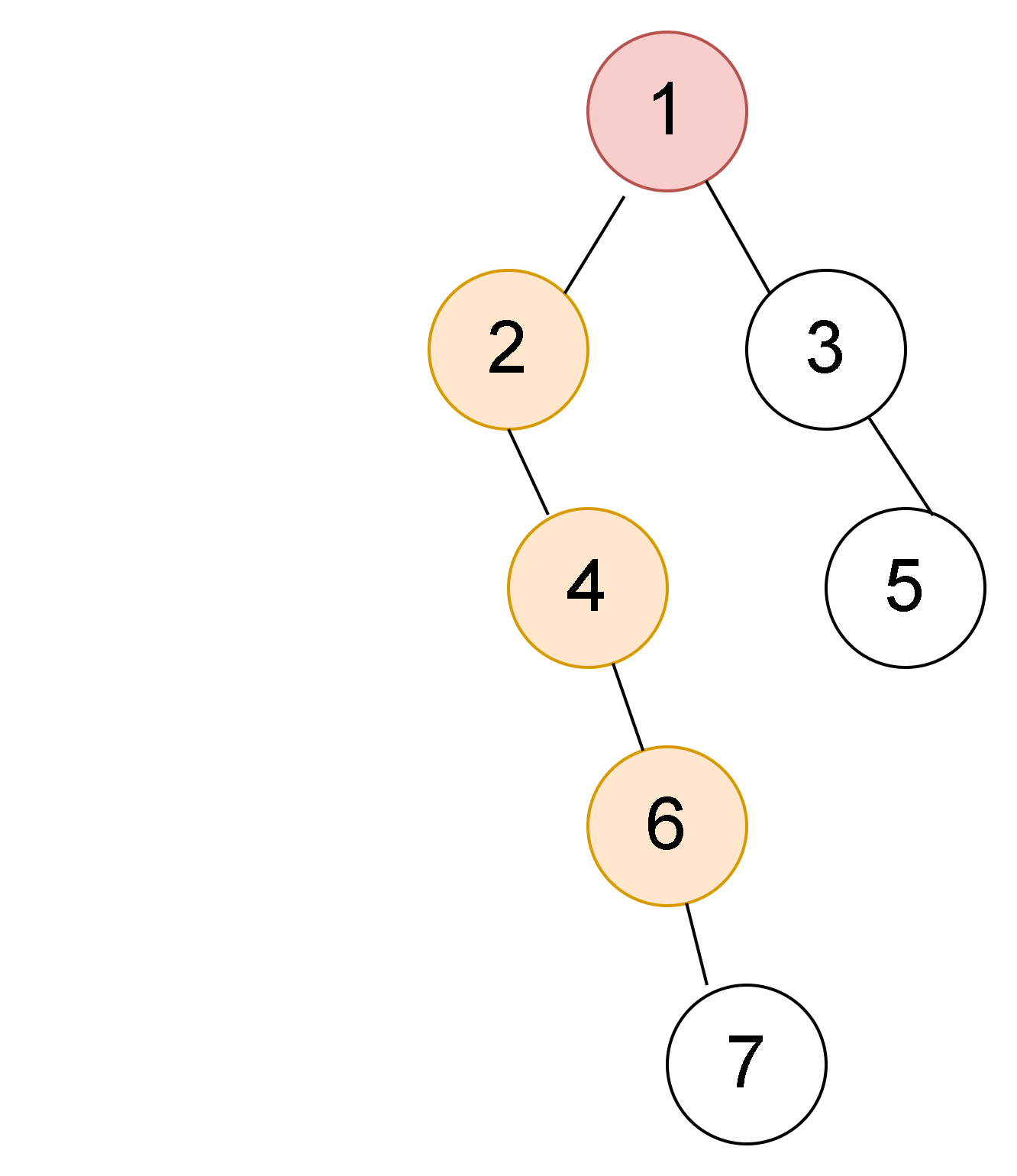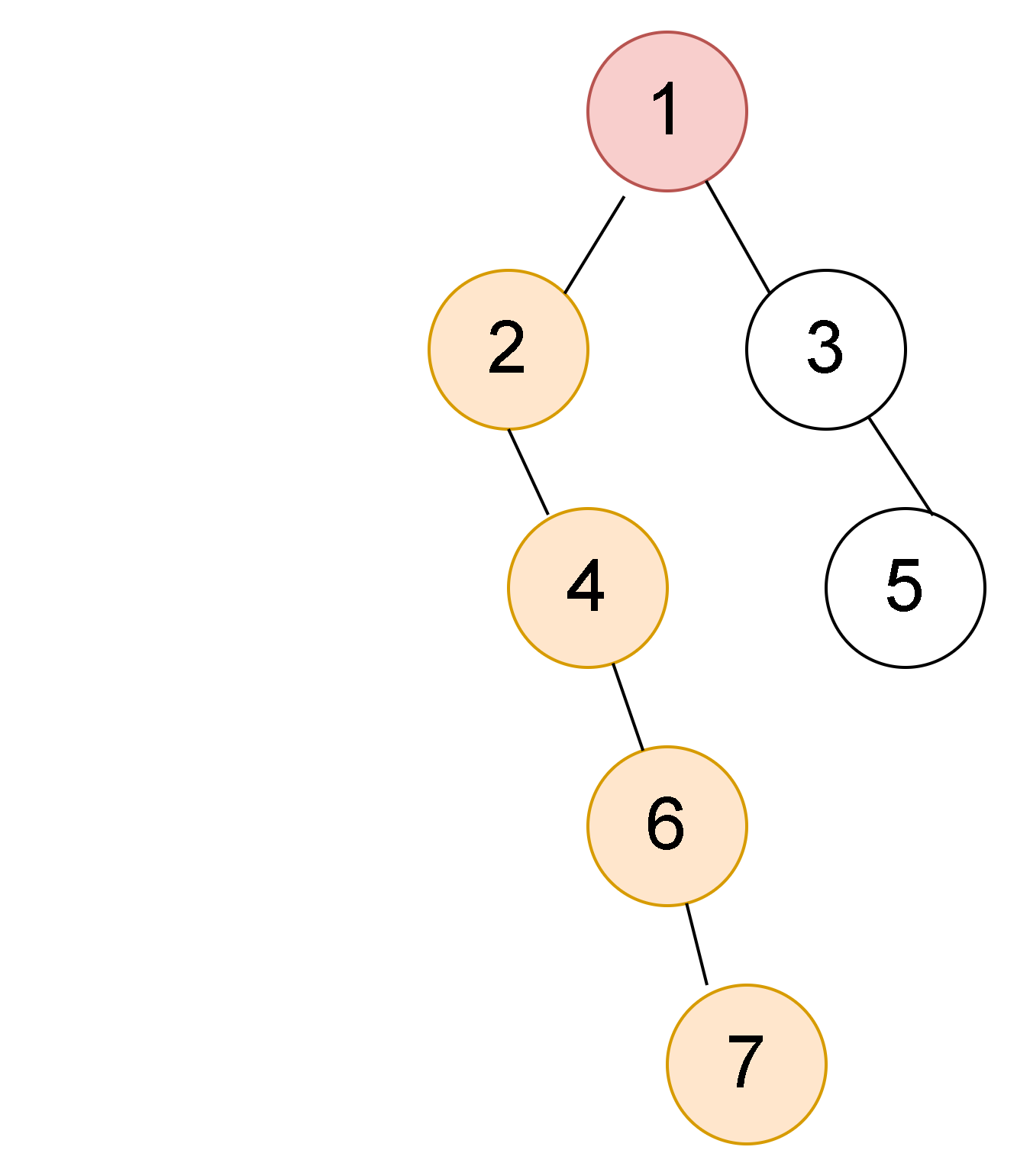If we carefully try to observe the  depth and height of 2, then as per the definition

Height - the height will be  4 (7->6->4->2)

Depth -  if we compute the depth then it will be 2 (1->2).

Now, let’s understand the approach behind this.

## Height of a Binary tree

First, let us understand the concept of the height of a binary tree. The height of a binary tree is the maximum distance from the root node to any leaf node. First, let's discuss the recursive approach to calculate the height of a binary tree.

1. We will start from the root node and initially, the height will be 0.
2. We will recursively calculate the height of the left subtree.
3. Similarly, we will calculate the height of the right subtree.
4. Now I will calculate the height of the current node by adding 1 to the max height between the right and left subtree.

## Algorithm

We can solve this problem using a recursive algorithm which will be used to traverse the binary tree. This traversal is similar to depth-first search in the graph algorithm. Let us understand this algorithm with the help of the code given below:

Output :

Time Complexity - O(N), as we are traversing every node once, the time complexity to compute the height of a binary tree is of the order N, where N is the number of nodes present in the tree.

Space Complexity -The space complexity is O(H), where H is the height of the binary tree considering the stack space used during recursion while checking for symmetric subtrees. In the worst case(skewed binary tree), H can be equal to N, where N is the number of nodes in the tree.

This problem given above can be modified as - Given the node’s address, we have to find the height of that particular node in the tree. We can simply find the given node and start traversing to the left and right. The function to compute the following is :

## Modified Height of a binary tree

Output :

Time Complexity - O(N), as we are traversing every node once, the time complexity to compute the height of a binary tree is N, where N is the number of nodes present in the tree.

Space Complexity - The space complexity is O(H), where H is the height of the binary tree considering the stack space used during recursion while checking for symmetric subtrees. In the worst case(skewed binary tree), H can be equal to N, where N is the number of nodes in the tree.

## Depth of a node in a binary tree

Now let us calculate the depth of a node in a binary tree -
We can simply calculate the depth of the tree by subtracting the height of the given node by the height of the root node. i.e -

Height(root) - Height(given node)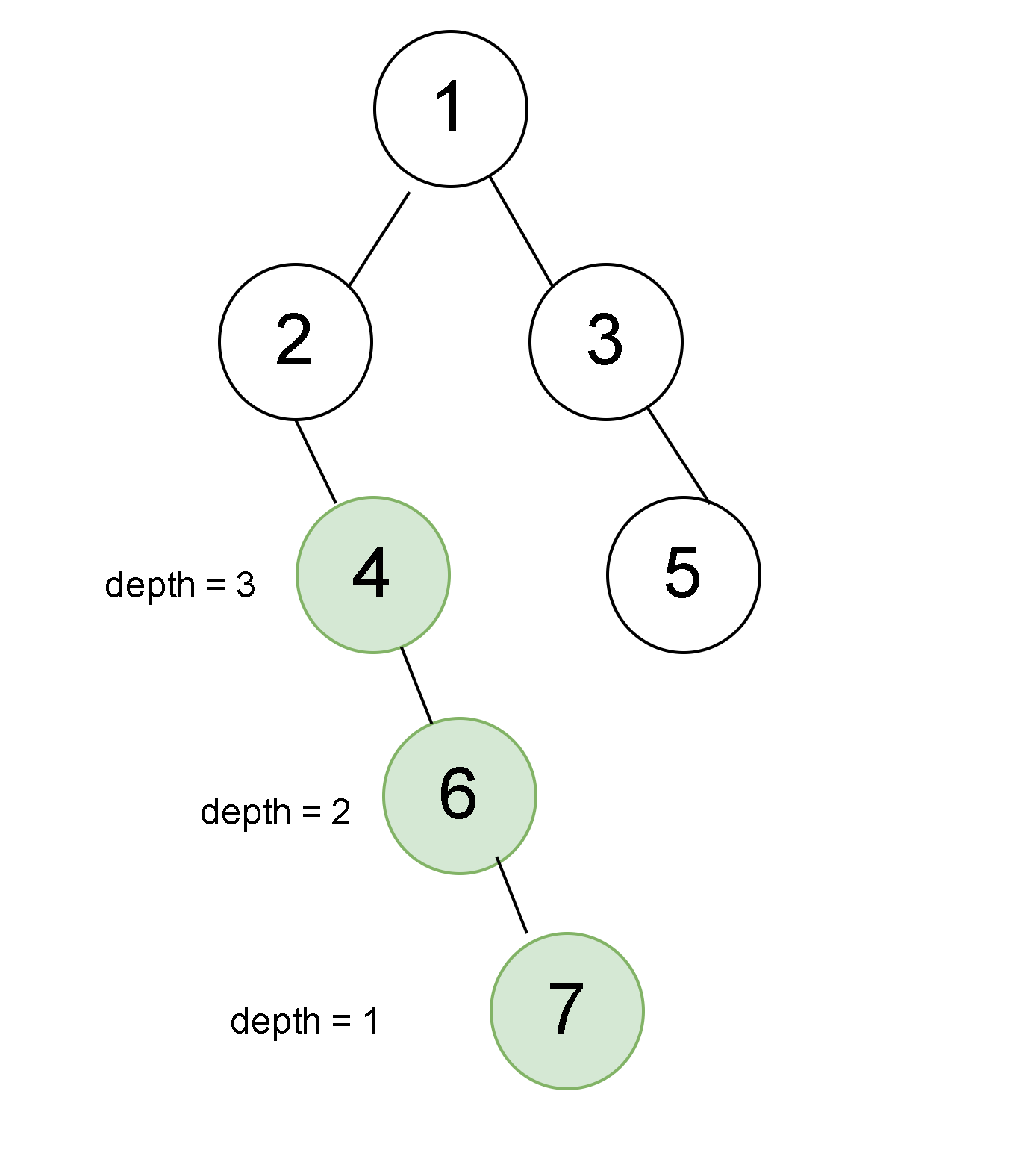We can also calculate the depth of the binary tree by applying the recursive algorithm. We initiate the depth to be 0 initially when we are present at the root node. On every recursive call we add one to the depth and return when the given element for which depth has to be calculated is equal to the current element.

Output :

Time Complexity - O(N), as we are traversing every node once, the time complexity to compute the height of a binary tree is N, where N is the number of nodes present in the tree. In the worst case our node will be leaf node and we will end up traversing all the nodes.

Space Complexity - The space complexity is O(H), where H is the height of the binary tree considering the stack space used during recursion while checking for symmetric subtrees. In the worst case(skewed binary tree), H can be equal to N, where N is the number of nodes in the tree.

## FAQs

Q1) What is a binary search tree?

A1) A binary Search Tree or BST is a tree used to sort, retrieve, and search data. It is also a non-linear data structure in which the nodes are arranged in a particular order.

• The left subtree of a node has nodes that are only lesser than that node’s key.
• The right subtree of a node has nodes that are only greater than that node’s key.
• Each node has distinct keys, and duplicates are not allowed in the Binary Search Tree.
• The left and right subtree must also be a binary search tree.

Q2) Is DFS & preorder traversal the same?

Preorder traversal is another variant of DFS. Depth-first-search (DFS) traverses the search tree as much as possible before proceeding to the next sibling, which is similar to pre-order traversal.

## Key Takeaways

In this article, we discussed the solution to achieve the height/depth of a binary tree. This is an easy recursive problem on binary trees and can be solved using a simple application of recursion. This concept is a very important topic and has application in other questions for binary tree data structure. You can also refer to our course on master tree data structure to understand Trees.